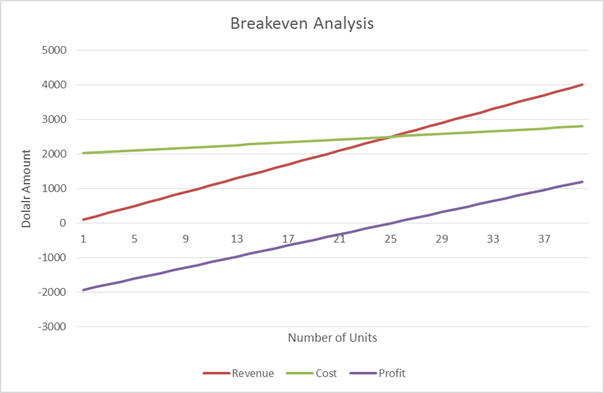Breakeven Analysis is used to calculate the number of units you need to sell in order to cover all of your fixed and variable costs.

Equation

Break Even Analysis:

Fixed Costs / (Price per Unit – Cost per Unit) = Quantity to Produce

Quantity to Produce (Price per Unit – Cost per Unit) – Fixed Cost = Amount Earned

Example

• Item A is sold for \$100 per unit.
• Item A costs \$20 per unit
• Company X has fixed costs of \$2,000

The breakeven point is:

\$2,000 / (\$100 – \$20) = 25 units